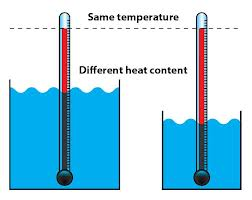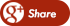﻿ WHET IS THE DIFFERENCE BETWEEN HEAT AND TEMPERATURE?Back

## WHET IS THE DIFFERENCE BETWEEN HEAT AND TEMPERATURE?

By Admin onThere is a fundamental difference between temperature and heat. Heat is the amount of energy in a system. The SI units for heat are Joules. A Joule is a Newton times a meter. A Newton is a kilogram-meter per second squared. Heat is transferred through radiation, conduction and convection. The amount that molecules are vibrating, rotating or moving is a direct function of the heat content. Energy is transported by conduction as molecules vibrate, rotate and/or collide into each other. Heat is moved along similar to dominos knocking down their neighbours in a chain reaction. An increase of electromagnetic radiation into a system causes the molecules to vibrate, rotate and/or move faster. With convection, higher energy molecules are mixed with lower energy molecules. When higher energy molecules are mixed with lower energy molecules the molecular motion will come into equilibrium over time. The faster moving molecules will slow down and the slow moving molecules will speed up.Temperature is the MEASURE of the AVERAGE molecular motions in a system and simply has units of (degrees F,  degrees C, or K). Notice that one primary difference between heat and temperature is that heat has units of Joules and temperature has units of (degrees F, degrees C, or K). Another primary difference is that energy can be transported without the temperature of a substance changing (e.g. latent heat, ice water remains at the freezing point even as energy is brought into the ice water to melt more ice). But, as a general statement (ignoring latent heat), as heat energy increases, the temperature will increase. If molecules increase in vibration, rotation or forward motion and pass that energy to neighbouring molecules, the measured temperature of the system will increase.

Thermal energy is the total of kinetic energy.Temperature is a measure of average heat.Heat is the average of kinetic energy